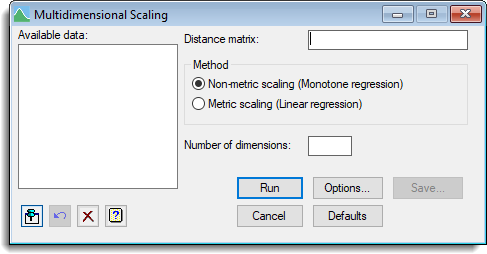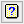1. Home
2. Multidimensional Scaling

# Multidimensional Scaling

Select menu: Stats | Multivariate Analysis | Multidimensional Scaling

Multidimensional scaling operates on a symmetric matrix whose values may be interpreted, in a general sense, as distances between a set of objects. The objective is to find a set of coordinates whose inter-point distances match, as closely as possible, those of the input data matrix. When plotted, the coordinates provide a display which can be interpreted in the same way as a map: for example, if points in the display are close together, their distance apart in the data matrix was small.

Stats | Multivariate Analysis | Multidimensional Scaling.
2. Fill in the fields as required then click Run.

You can set additional Options then after running, you can save the results by clicking Save.## Available data

This lists symmetric matrices that can be used to specify the distance data. Double-click a name to copy it to the current input field or type the name.

## Distance matrix

Specifies the symmetric matrix of inter-point distances.

## Method

Controls whether metric or non-metric scaling is given. The algorithm involves regression of the distances, calculated from the solution coordinates, against the distances in the data matrix. Non-metric scaling uses monotonic regression, whereas metric scaling uses linear regression through the origin.

## Number of dimensions

Sets the number of dimensions required for the solution. Entering a list of numbers carries out a series of scaling operations, all based on the same matrix of dissimilarities, but with different numbers of dimensions.

## Options

You can control various aspects of the algorithm used for the analysis from the Options menu, and also select which results are to be printed.

## Action IconsPin Controls whether to keep the dialog open when you click Run. When the pin is downthe dialog will remain open, otherwise when the pin is upthe dialog will close.Restore Restore names into edit fields and default settings.Clear Clear all fields and list boxes.Help Open the Help topic for this dialog.